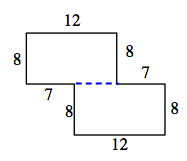### Home > CAAC > Chapter 2 > Lesson 2.1.1 > Problem2-8

2-8.
1. Copy the following figures onto your paper. Then find the area and perimeter of each shape. Assume that all corners are right angles. Show all work. Homework Help ✎

1.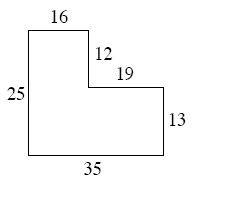2.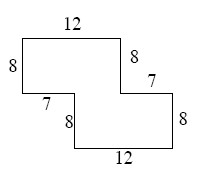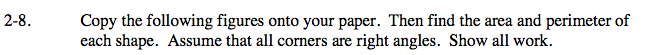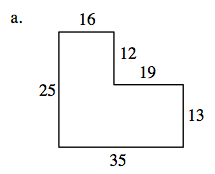To find the area, separate the shape into two boxes, find the area of the two sections, and then add them together for the total area.

16 x 12 = 192
13 x 35 = 455
area = 192 + 455

area = 647 units2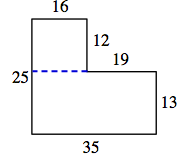To find the perimeter (the distance around a shape) add up all of the sides of the shape.

perimeter = 16 + 12 + 19 + 13 + 35 + 25

perimeter = 120 units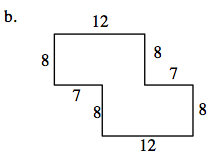To find the area and perimeter of the shape in part (b), follow the same strategy outlined in part (a).